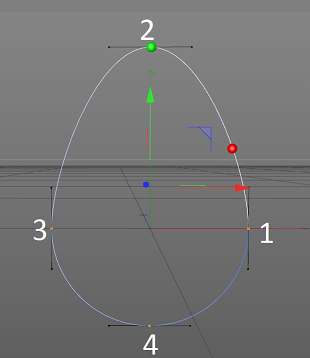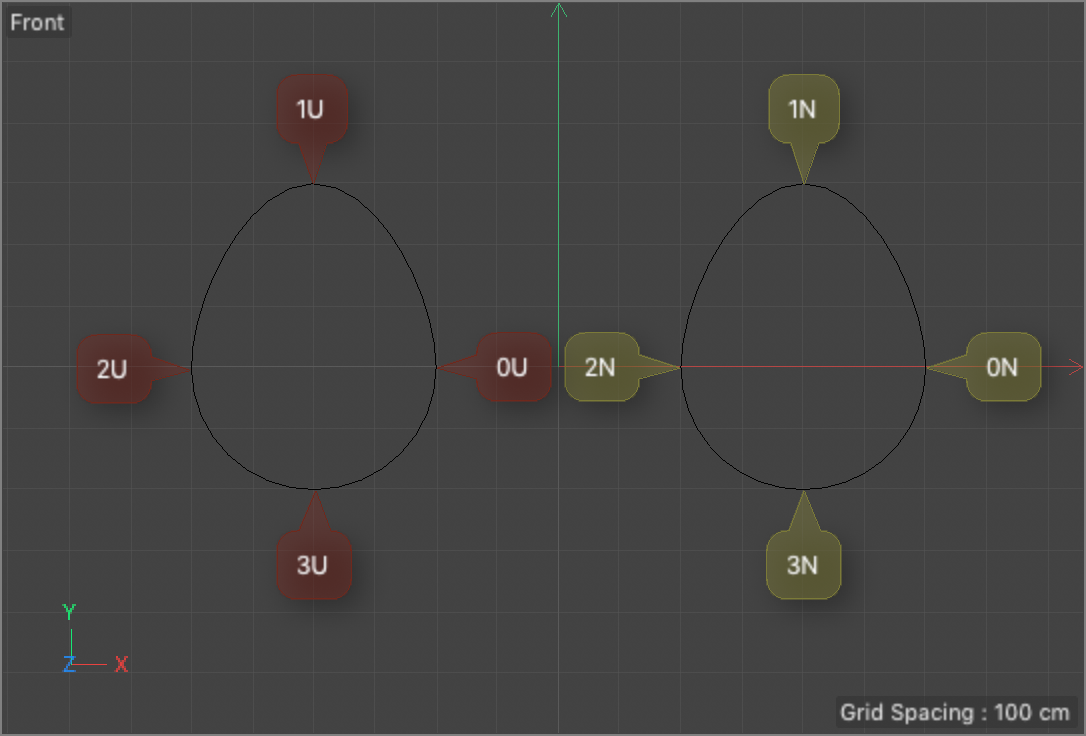# GetSplinePointSegment Offset

• Hello,

Using the SplineHelp class I am attempting to get the percentage offset along a spline segment of each point on that spline. Based off its description in the SDK the function GetSplinePointSegment would give me the offset value that I'm looking for.

My issue comes in when I cycle through the points of the spline and feed the spline point index into GetSplinePointSegment it usually returns evenly distributed offset values despite the fact that the spline I'm using does not have an even distribution of its points along its length.

This is the code I am using to utilize GetSplinePointSegment.

``````Int32 pointCount = spline->GetPointCount();
for (Int32 pointIndex = 0; pointIndex < pointCount; pointIndex++)
{
Int32 index = 0;
Int32 segment = 0;
Float offset = 0;
helperSpline->GetSplinePointSegment(pointIndex, &offset, &index, &segment);

ApplicationOutput("Point data " + String::IntToString(pointIndex) + "    " + String::FloatToString(offset) + "   " + String::IntToString(index) + "  " + String::IntToString(segment));
}
``````

When I feed in a circle spline with four points that are evenly distributed GetSplinePointSegment returns 25% from the offset variable. If I move one of the points out of its place the offset variable does not properly adjust to reflect this.

For the purposes of what I'm working on I need the accurate offset percentage of the various points along a spline and I seem to be missing something fundamental about how SplineHelp works. I looked through the forum and saw some other posts dealing with a similar issue from a while ago but the solutions proposed in those posts don't work in my code.

Some help on understanding why GetSplinePointSegment is returning the values it is, or a suggestion of which function would give me the information I need would be greatly appreciated.

John Thomas

• Hello,

Cheers,
Manuel

• Thanks for the response.

I looked over the thread and from what I understand,

GetOffsetFromReal will provide you with an offset in spline space when you give it an offset value between 0 and 1.
GetOffsetFromUnit gives you the natural offset when you give it a distance between 0 and the length of the segment.

This is the spline I've been using to run tests on.When I use GetSplinePointSegment to retrieve the offset for the second point of the spline it gives me an offset of .222, I feed that offset value into GetPosition (return value represented by the green sphere). Feeding the offset of .222 into GetOffsetFromReal returns an offset value of .11 which when used in GetPosition provides the position of the red sphere. Through separate testing I've confirmed that the second point is roughly 30% of the way along the length of the spline which is the kind of return value I am looking for.

I'm looking to get the distance along the spline for each of the points individually. In the example above if the spline has a length of 1500 (Based of the positions of the points I would expect their offset to be 0, .3, .6, and .8 respectively) I would expect the distance from point 1 to point 2 to be about 450, another 450 from point 2 to 3 and so on.

John Thomas

• Hi @JohnThomas, thanks for following up.

I've a few points here:

• the curve parametrization matters: depending on having it set to natural or uniform you get different results:
• Natural: given a certain percentage [0:1], `GetPosition()` and `GetSplinePoint()` returns the same value - viceversa passing the same percentage to `GetOffsetFromReal()` returns the offset value that you should use with the uniform parametrization to get the same point position
• Uniform: given a certain percentage [0:1], `GetPosition()` and `GetSplinePoint()` don't return the same value - viceversa passing the same percentage to `GetOffsetFromReal()` returns the same value (excluding rounding errors).
• `GetOffsetFromReal()`: accept an input in [0:1] and returns the natural percentage/offset/parametrization given a uniform percentage/offset/parametrization()
• `GetOffsetFromUnit()`: accept an input in [0:the length of the spline] and return the uniform percentage/offset/parametrization for that length.

Now coming to your question of getting the distance of each point from the origin: if your spline is using a uniform parametrization since offset and offsetFromReal are equal you can use the offset value returned by `GetSplinePointSegment()` for each point and multiplying it by the whole length of the spline you get the distance of that point from the spline origin.
If your spline instead is natural, since the natural mode only cares about the interpolation of the curve parameter, all the curve segments (1-2 , 2-3, 3-4, 4-1) are of the same length equal to 1/4 of the whole length.

Finally I recommend to have a look at the SplineLengthData class which provide the convenient UniformToNatural method.

Best, Riccardo

• Thanks for the detailed response.

I changed the spline to uniform to test and I'm confused about the results being returned from GetSplinePointSegment(). From the spline example above I am now getting an offset of 0.278 from point 2, 0.583 from point 3, and 0.778 from point 4 using the code below.

``````Int32 pointCount = spline->GetPointCount();
for (Int32 pointIndex = 0; pointIndex < pointCount; pointIndex++)
{
Int32 index = 0;
Int32 segment = 0;
Float offset = 0;

helperSpline->GetSplinePointSegment(pointIndex, &offset, &index, &segment);
}
``````

Given the points of the spline I would have expected point 1 and 2 to have an equal increase in their offsets.

John Thomas

• Hi @JohnThomas thanks for following up.

In the image below there are two deformed circles (radius 200) where the one on the left has a uniform parametrization whilst the right a natural one.Both curves have an overall length of 1403.691 cm and are made up of 1 segment and 4 points.

For the natural-parametrized spline, the GetSplinePointSegment() returns for points 0N, 1N, 2N, and 3N respectively the values of 0.0, 0.25, 0.5 and 0.75 to which correspond the following lengths 0.0, 350.923, 701.846, 1052.768 and which given that kind of parametrization are right because they are all equal.

For the uniform-parametrized spline, the GetSplinePointSegment() returns for points 0N, 1N, 2N, and 3N respectively the values of 0.0, 0.278, 0.556 and 0.778 to which correspond the following lengths 0.0, 389.871, 779.742, 1091.639. These values are correct and this is confirmed by the fact that delta between 0U-1U is 389.871 as well as 1U-2U (389.871) whilst 2U-3U and 3U-0U are both (311.897).

Finally when the SplineLengthData class is used on the "uniform" curve to "transform" the offset values to the natural one the tolerance (Offset delta) are pretty low

``````Natural [4, 1403.691, BEZIER, 1]
0N [0/0], 0.000[0.000]
1N [9/0], 0.250[350.923]
2N [18/0], 0.500[701.846]
3N [27/0], 0.750[1052.768]

Uniform [4, 1403.536, BEZIER, 2]
0U [0/0], 0.000[0.000] --> 0.000[0.000]
1U [10/0], 0.278[389.871] --> 0.251[352.940] / Offset delta [0.001]
2U [20/0], 0.556[779.742] --> 0.503[705.883] / Offset delta [0.003]
3U [28/0], 0.778[1091.639] --> 0.751[1054.710] / Offset delta [0.001]
``````

Best, Riccardo

• Thanks for the detailed response.

I mimicked your setup for the uniform spline and I am getting the same output from GetSplinePointSegment(). When I take the offset from GetSplinePointSegment() and use it in GetPosition() (just to check if the position matches that of the point the returned position) the returned position does not match the equivalent point on the spline.

If I deform the circle spline more this behavior continues and the vector form GetPosition() is further from the position it should be.

John Thomas

• Hi @JohnThomas thanks for the follow-up.

I see the point, but when you're dealing with uniform splines you've also to consider the number of intermediate points you're using to approximate the natural spline. With a natural parametrization even with a low number of points the vertexes position returned from SplineHelp::GetPosition() are accurate, whilst with a uniform parametrization you get higher accuracy as long as you increase the number of points.

See the data below, where first coordinate is the position returned by the SplineHelp::GetPosition and the second coordinate is returned by quering the points vector PointsObject::GetPointR()

``````Natural_4 [4, 2123.461, BEZIER, 1]
0 [0/0], 0.000[0.000] -->  (200.000,0.000,0.000) -- (200.000,0.000,0.000)
1 [17/0], 0.250[530.865] -->  (0.000,700.000,0.000) -- (0.000,700.000,0.000)
2 [34/0], 0.500[1061.731] -->  (-200.000,0.000,0.000) -- (-200.000,0.000,0.000)
3 [51/0], 0.750[1592.596] -->  (0.000,-200.000,0.000) -- (0.000,-200.000,0.000)

Uniform_4 [4, 2107.332, BEZIER, 2]
0 [0/0], 0.000[0.000] --> (200.000,0.000,0.000) -- (200.000,0.000,0.000)
1 [7/0], 0.350[737.566] --> (4.370,699.695,0.000) --> (0.000,700.000,0.000)
2 [14/0], 0.700[1475.132] --> (-199.849,8.692,0.000) -- (-200.000,0.000,0.000)
3 [17/0], 0.850[1791.232] --> (-4.394,-199.953,0.000) -- (0.000,-200.000,0.000)

Uniform_16 [4, 2107.332, BEZIER, 2]
0 [0/0], 0.000[0.000] --> (200.000,0.000,0.000) -- (200.000,0.000,0.000)
1 [7/0], 0.350[737.566] --> (4.370,699.695,0.000) -- (0.000,700.000,0.000)
2 [14/0], 0.700[1475.132] --> (-199.849,8.692,0.000) -- (-200.000,0.000,0.000)
3 [17/0], 0.850[1791.232] --> (-4.394,-199.953,0.000) -- (0.000,-200.000,0.000)

Uniform_128 [4, 2124.555, BEZIER, 2]
0 [0/0], 0.000[0.000] --> (200.000,0.000,0.000) -- (200.000,0.000,0.000)
1 [182/0], 0.353[749.359] --> (-1.373,699.970,0.000) -- (0.000,700.000,0.000)
2 [363/0], 0.703[1494.600] --> (-199.996,1.279,0.000) -- (-200.000,0.000,0.000)
3 [440/0], 0.853[1811.636] --> (1.380,-199.995,0.000) -- (0.000,-200.000,0.000)
``````

If no further help is needed please don't forget to set the thread as Solved.

Cheers, R